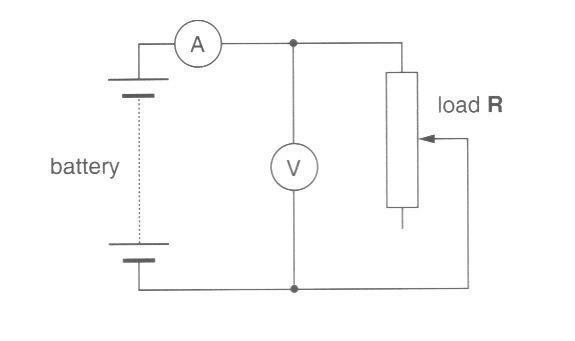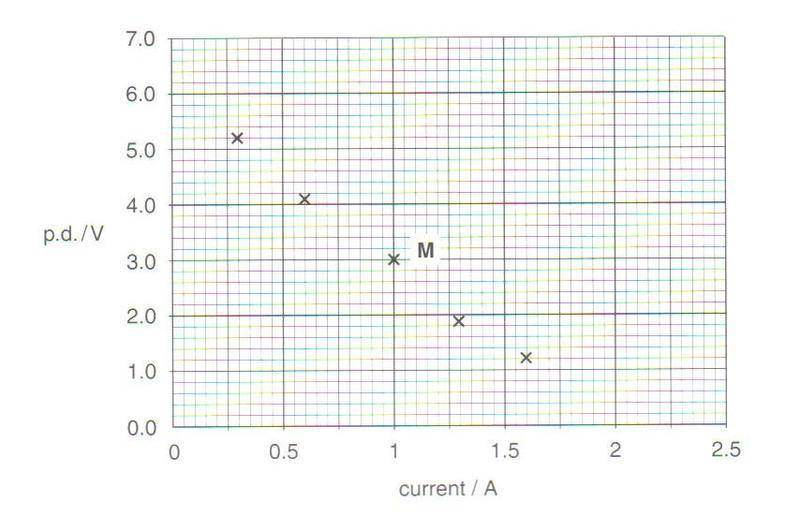# General help understanding emf and p.d

• Gregg
In summary, a graph is used to show the variation of potential difference (V) across a battery with current (I) as the resistance (R) is changed. A line of best fit is drawn and its gradient is used to determine the emf of the battery and its internal resistance (r). The equation E=V+Ir is used to find the emf, and by rearranging it in the form of a straight line, the y-intercept represents the emf and the gradient represents the internal resistance. This concept is similar to the graph of an ohmic conductor, where V and I are directly proportional.

#### GreggGraph to show the variation of the p.d. V across the battery with current I as R is varied.i) Draw line of best fit.

ii) Use it to determine the emf of the battery;

and the internal resistance r of the battery.

The gradient $\approx \frac{\Delta V}{\Delta I} = 1.67 \Omega$

Just don't know what to do really. Need an example and explanation of the concept.

Let the internal resistance of the battery be r.
The same current,I, passes through both r and R right?

So by Kirchoff's 2nd law

the emf of the battery=sum of the pd's around the loop
If V is the pd across the load then

E=V+Ir.

Since they plotted V against I, rearrange the equation it in the form a straight line y=mx+c

you calculated the gradient correctly to give a resistance. So rearrange the equation and you will see what that gradient represents and what the intercept represents as well.

$V = f(I)$

$V = -Ir + E$

y-intercept is emf, gradient is -r. Thanks.

Hi I had a similar question, which I posted at the the thread 'internal resistance graph'. It was basically why are V are I inversely proportional in this graph, but proportional in the graph of an ohmic conductor?